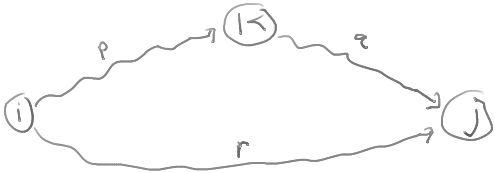# TransitiveClosureByDP

ClassS04CS141 | recent changes | Preferences

#### Transitive Closure

(Read section 6.4.2 of the text.)

Given a digraph G = (V,E), we want to compute a matrix M such that M[i,j] is true if there is a path from i to j.

One way is to iterate over all vertices and do a depth-first or breadth-first search starting from each vertex to see what vertices are reachable from it. This takes O(n(n+m)) time.

Here's another more complicated way.

Order the vertices v1,v2,...,vn in some arbitrary way.

Define M[i,j,k] to be true if there is a path from i to j that goes through only the first k vertices v1,...,vk. (That is, i and j need not be in the set of k vertices, but all the other vertices on the path must be.)

For example:

M[i,j,0] = true if there is an edge from i to j in E.

M[i,j,1] = true if there is an edge from i to j or edges (i,1) and (1,j)

M[i,j,n] = true if there is any path from i to j.

Claim: M[i,j,k] = true iff (M[i,j,k-1] = true or (M[i,k,k-1] = true and M[k,j,k-1] = true) )

To see this, consider the diagram:Any path from i to j going only through vertices in the set {1,2,...,k} is of one of the following two forms:

1. The path goes from i to j using only vertices in the set {1,2,...,k-1}.
2. The path goes from i to k using only vertices in the set {1,2,...,k-1} and then goes from k to j using only vertices in th set {1,2,...,k-1}.

This recurrence leads to the following algorithm:

```  1. Initialize M[i,j] = false for all i,j.
2. Set M[i,j] = true for each edge (i,j) in E.
3. For k = 1,2,...,n
4.   For i = 1,2,...,n
5.     For j = 1,2,...,n
6.        M[i,j] = M[i,j] || (M[i,k,k-1] && M[k,j,k-1])
7. Return M.
```

The algorithm runs in n3 time.

This isn't particularly fast, however we will see later (ShortestPathsByDP) how to modify the algorithm to compute shortest paths (a slightly more difficult problem).

### References

ClassS04CS141 | recent changes | Preferences